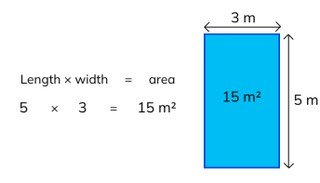Determining area of a rectangle

# Determining area of a rectangle

Students learn to determine area of a rectangle.8,000 schools use Gynzy92,000 teachers use Gynzy1,600,000 students use Gynzy

## General

The students learn to determine the area of a rectangle.

## Standards

CCSS.Math.Content.3.MD.C.5/6

## Learning objective

Students will be able to determine the area of a rectangle.

## Introduction

On the interactive whiteboard there are three rectangles in squares. The students determine the area by counting the squares or by solving a multiplication problem. Have the students explain how they calculated the area.

## Instruction

You ask if one of the students can explain how you determine area. Next you can show this using the image on the interactive whiteboard. After this you show how you determine the area of a rectangle through discussing how you can determine what problem matches the shape. Then explain the concept of square meters. Next the students practice finding the correct problems to match the shapes. You can write the letter of the shape next to the problem. Next you discuss several shapes for which the students must determine the area. They first come up with what problem they must make and then they solve it. At the end of the section you discuss how you can solve a story problem about area and solve the following story problems together with the students.

To check whether the students can determine area of a rectangle, you can ask the following questions:
- How can you determine area?
- What kind of problem can you make to determine area?
- What type of measurement do you have as the outcome of an area?

## Quiz

The students test their understanding of determining area of rectangles through ten exercises. For each of the exercises the students must determine the area of the shape. Some of the exercises are story problems.

## Closing

You repeat the learning goal and the importance of determining the area of a rectangle. Next there are five rectangles on the interactive whiteboard with the length and width of each one. In a thought bubble there are the areas of the rectangles. The students must determine which area belongs with which shape. You can drag the numbers from the thought bubble to the shapes. Have the students explain what problem they made to determine the area of each shape.

## Teaching tips

When students have difficulty with this learning goal, you can have them draw a shape for the story problems and label sides with their measurements.

## Instruction materials

Graphing paper

### The online teaching platform for interactive whiteboards and displays in schools

• Save time building lessons

• Manage the classroom more efficiently

• Increase student engagement